## From Fourier Analysis to Wavelet Analysis

### Inner Products

Both the Fourier and wavelet transforms measure similarity between a signal and an analyzing function. Both transforms use a mathematical tool called an inner product as this measure of similarity. The two transforms differ in their choice of analyzing function. This results in the different way the two transforms represent the signal and what kind of information can be extracted.

As a simple example of the inner product as a measure of similarity, consider the inner product of vectors in the plane. The following MATLAB® example calculates the inner product of three unit vectors, $\left\{u,v,w\right\}$, in the plane:

`$\begin{array}{l}\left\{\left(\begin{array}{c}\sqrt{3}/2\\ 1/2\end{array}\right),\left(\begin{array}{c}1/\sqrt{2}\\ 1/\sqrt{2}\end{array}\right),\left(\begin{array}{c}0\\ 1\end{array}\right)\right\}\\ \end{array}$`

```u = [sqrt(3)/2 1/2]; v = [1/sqrt(2) 1/sqrt(2)]; w = [0 1]; % Three unit vectors in the plane quiver([0 0 0],[0 0 0],[u(1) v(1) w(1)],[u(2) v(2) w(2)]); axis([-1 1 0 1]); text(-0.020,0.9371,'w'); text(0.6382,0.6623,'v'); text(0.7995,0.4751,'u'); % Compute inner products and print results fprintf('The inner product of u and v is %1.2f\n', dot(u,v)) fprintf('The inner product of v and w is %1.2f\n', dot(w,v)) fprintf('The inner product of u and w is %1.2f\n', dot(u,w))```

Looking at the figure, it is clear that u and v are most similar in their orientation, while u and w are the most dissimilar.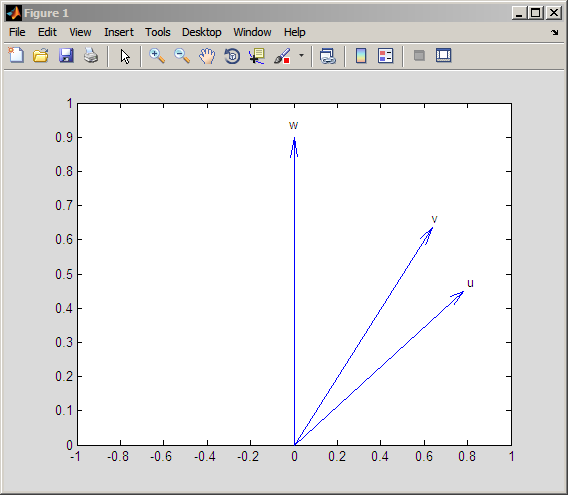The inner products capture this geometric fact. Mathematically, the inner product of two vectors, u and v is equal to the product of their norms and the cosine of the angle, θ, between them:

`$\begin{array}{l}=|\text{​}\text{ }|u|\text{ }||\text{ }|v|\text{ }|\mathrm{cos}\left(\theta \right)\\ \end{array}$`

For the special case when both u and v have unit norm, or unit energy, the inner product is equal to cos(θ) and therefore lies between [-1,1]. In this case, you can interpret the inner product directly as a correlation coefficient. If either u or v does not have unit norm, the inner product may exceed 1 in absolute value. However, the inner product still depends on the cosine of the angle between the two vectors making it interpretable as a kind of correlation. Note that the absolute value of the inner product is largest when the angle between them is either 0 or $\pi$ radians (0 or 180 degrees). This occurs when one vector is a real-valued scalar multiple of the other.

While inner products in higher-dimensional spaces like those encountered in the Fourier and wavelet transforms do not exhibit the same ease of geometric interpretation as the previous example, they measure similarity in the same way. A significant part of the utility of these transforms is that they essentially summarize the correlation between the signal and some basic functions with certain physical properties, like frequency, scale, or position. By summarizing the signal in these constituent parts, we are able to better understand the mechanisms that produced the signal.

### Fourier Transform

Fourier analysis is used as a starting point to introduce the wavelet transforms, and as a benchmark to demonstrate cases where wavelet analysis provides a more useful characterization of signals than Fourier analysis.

Mathematically, the process of Fourier analysis is represented by the Fourier transform:

`$F\left(\omega \right)={\int }_{-\infty }^{\infty }f\left(t\right){e}^{-j\omega t}dt.$`

which is the integral (sum) over all time of the signal f(t) multiplied by a complex exponential. Recall that a complex exponential can be broken down into real and imaginary sinusoidal components. Note that the Fourier transform maps a function of a single variable into another function of a single variable.

The integral defining the Fourier transform is an inner product. See Inner Products for an example of how inner products measure of similarity between two signals. For each value of ω, the integral (or sum) over all values of time produces a scalar, F(ω), that summarizes how similar the two signals are. These complex-valued scalars are the Fourier coefficients. Conceptually, multiplying each Fourier coefficient, F(ω), by a complex exponential (sinusoid) of frequency ω yields the constituent sinusoidal components of the original signal. Graphically, the process looks like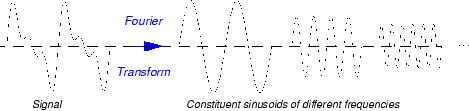Because ${e}^{j\omega t}$ is complex-valued, F(ω) is, in general, complex-valued. If the signal contains significant oscillations at an angular frequency of ${\omega }_{0}$, the absolute value of $F\left({\omega }_{0}\right)$ will be large. By examining a plot of $|F\left(\omega \right)|$ as a function of angular frequency, it is possible to determine what frequencies contribute most to the variability of f(t).

To illustrate how the Fourier transform captures similarity between a signal and sinusoids of different frequencies, the following MATLAB code analyzes a signal consisting of two sinusoids of 4 and 8 Hertz (Hz) corrupted by additive noise using the discrete Fourier transform.

```rng(0,'twister'); Fs = 128; t = linspace(0,1,128); x = 2*cos(2*pi*4*t)+1.5*sin(2*pi*8*t)+randn(size(t)); xDFT = fft(x); Freq = 0:64; subplot(211); plot(t,x); xlabel('Seconds'); ylabel('Amplitude'); subplot(212); plot(Freq,abs(xDFT(1:length(xDFT)/2+1))) set(gca,'xtick',[4:4:64]); xlabel('Hz'); ylabel('Magnitude'); ```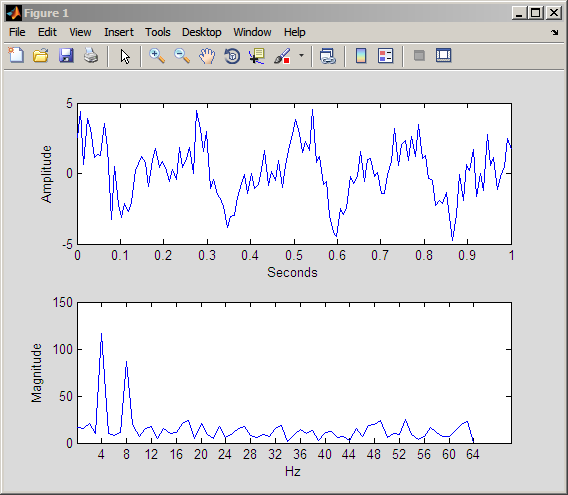Viewed as a time signal, it is difficult to determine what significant oscillations are present in the data. However, looking at the absolute value of the Fourier transform coefficients as function of frequency, the dominant oscillations at 4 and 8 Hz are easy to detect.

### Short-Time Fourier Transform

The Fourier transform summarizes the similarity between a signal and a sinusoid with a single complex number. The magnitude of the complex number captures the degree to which oscillations at a particular frequency contribute to the signal's energy, while the argument of the complex number captures phase information. Note that the Fourier coefficients have no time dependence. The Fourier coefficients are obtained by integrating, or summing, over all time, so it is clear that this information is lost. Consider the following two signals: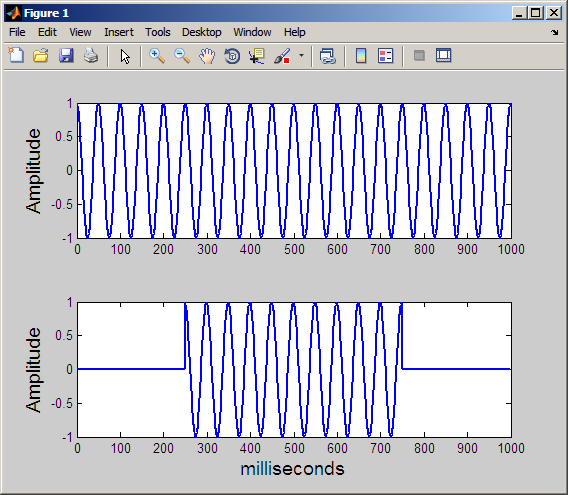Both signals consist of a single sine wave with a frequency of 20 Hz. However, in the top signal, the sine wave lasts the entire 1000 milliseconds. In the bottom plot, the sine wave starts at 250 and ends at 750 milliseconds. The Fourier transform detects that the two signals have the same frequency content, but has no way of capturing that the duration of the 20 Hz oscillation differs between the two signals. Further, the Fourier transform has no mechanism for marking the beginning and end of the intermittent sine wave.

In an effort to correct this deficiency, Dennis Gabor (1946) adapted the Fourier transform to analyze only a small section of the signal at a time -- a technique called windowing the signal. Gabor's adaptation is called the short-time Fourier transform (STFT). The technique works by choosing a time function, or window, that is essentially nonzero only on a finite interval. As one example consider the following Gaussian window function:

`$w\left(t\right)=\sqrt{\frac{\alpha }{\pi }}{e}^{-\alpha {t}^{2}}$`

The Gaussian function is centered around t=0 on an interval that depends on the value of α. Shifting the Gaussian function by τ results in:

`$w\left(t-\tau \right)=\sqrt{\frac{\alpha }{\pi }}{e}^{-\alpha {\left(t-\tau \right)}^{2}},$`

which centers the Gaussian window around τ. Multiplying a signal by $w\left(t-\tau \right)$ selects a portion of the signal centered at τ. Taking the Fourier transform of these windowed segments for different values of τ, produces the STFT. Mathematically, this is:

`$F\left(\omega ,\tau \right)=\int f\left(t\right)w\left(t-\tau \right){e}^{-j\omega t}dt$`

The STFT maps a function of one variable into a function of two variables, ω and τ. This 2-D representation of a 1-D signal means that there is redundancy in the STFT. The following figure demonstrates how the STFT maps a signal into a time-frequency representation.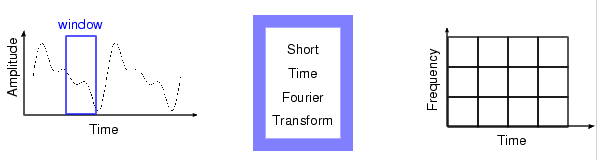The STFT represents a sort of compromise between time- and frequency-based views of a signal. It provides some information about both when and at what frequencies a signal event occurs. However, you can only obtain this information with limited precision, and that precision is determined by the size of the window.

While the STFT compromise between time and frequency information can be useful, the drawback is that once you choose a particular size for the time window, that window is the same for all frequencies. Many signals require a more flexible approach -- one where you can vary the window size to determine more accurately either time or frequency.

Instead of plotting the STFT in three dimensions, the convention is to code $|F\left(\omega ,\tau \right)|$ as intensity on some color map. Computing and displaying the STFT of the two 20-Hz sine waves of different duration shown previously: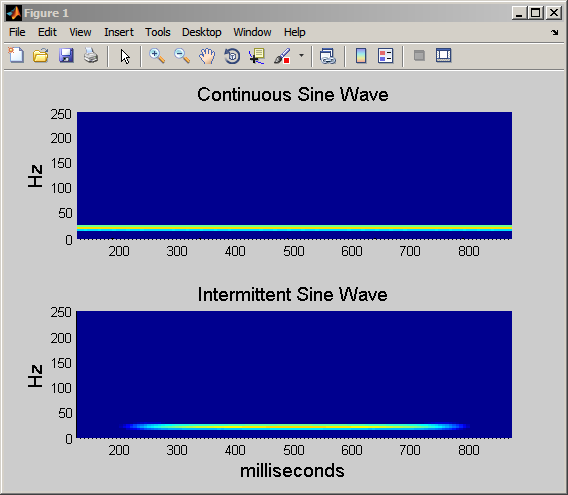By using the STFT, you can see that the intermittent sine wave begins near 250 msec and ends around 750 msec. Additionally, you can see that the signal's energy is concentrated around 20 Hz.

## SupportGet trial now